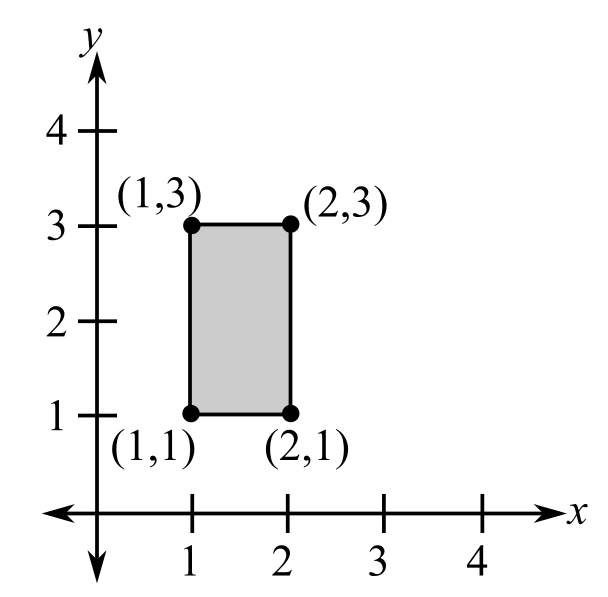Chapter 7.8, Problem 26E### Calculus: An Applied Approach (Min...

10th Edition
Ron Larson
ISBN: 9781305860919

#### Solutions

Chapter
Section### Calculus: An Applied Approach (Min...

10th Edition
Ron Larson
ISBN: 9781305860919
Textbook Problem
1 views

# Finding Area with a Double Integral In Exercises 25-30, use a double integral to find the area of the specified region. See Example 3.To determine

To calculate: The area of region by using double integration shown below.Explanation

Given Information:

The provided region is.

Formula used:

If a region is R defined in the domain of ayb and cxd, then,

The area of the region R is,

A=cdabdydx

Calculation:

Consider the region,

The bound for x are 1x2 and the bound for y are 1y3.

The area of the region is

A=1213dydx

Integrate with respect to y by holding x constant,

1213dydx=

### Still sussing out bartleby?

Check out a sample textbook solution.

See a sample solution

#### The Solution to Your Study Problems

Bartleby provides explanations to thousands of textbook problems written by our experts, many with advanced degrees!

Get Started

#### Find more solutions based on key concepts